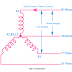The terms Line and Phase come in AC or Alternating power supply system. Especially, in the three-phase electrical power system, star and delta connection use these terms. You must know about these terms to understand an electrical balance system and an unbalanced system. Voltage is the potential difference across a conductor that actually forces to start the flow of current on the other hand current is the flow of electrons through a conductor.

There are two types of connections that are used in a three-phase power system - 1. Star Connection 2. Delta Connection.

Star Connection is that where one terminal of all the three phases is connected to a neutral point. The delta connection is that where all the three phases are connected in a closed-loop system, the ending of one phase is connected to the starting of another phase. Here, you can see the diagram of both Star and Delta Connection.

## What is Line Voltage?

In a three-phase system, the potential difference or voltage between any two phases or live terminal is called Line Voltage. So, if the three phases are R, Y, B then the line voltage can get across R-Y or Y-B or B-R. Line Voltage is denoted by VL. Generally, the line voltage is the higher voltage of a three-phase power system. Line voltage is available at 440V in India. Line voltage is greater than phase voltage.

In a star-connected power system, the line voltage is root three(3) times higher than the phase voltage. So, VL = 3*VPh or VL = 1.732VPh

In a delta-connected power system, the line voltage is equal to the phase voltage. So, VL = VPh

Line Voltage Example: 33KV, 11KV, and 440V are examples of line voltage. 440V is mostly used for consumer power supply and other voltages are used for power transmission.

## What is Phase Voltage?

In a power system, the potential difference or voltage between any one phase and the neutral point is called Phase Voltage. The phase voltage is denoted by VPh. If the three phases are - R, Y, and B and the neutral point is N, then the phase voltage can get across R-N or Y-N or B-N. In a power system, phase voltage comes with 230V in India. Phase voltage is lower than the Line Voltage.

In a star-connected power system, the phase voltage VPh = VL/3

In a delta-connected power system, the phase voltage is equal to the line voltage or VPh = VL

Phase Voltage Example: 230V single-phase supply is an example of phase voltage.

## Difference Between Line Voltage and Phase Voltage

1. Line Voltage is high voltage whereas Phase voltage is low voltage.

2. To get Line Voltage both live terminals are required whereas to get phase voltage any one phase terminal is required.

## What is Line Current?

The flow of current through any one line between the three-phase power source and load is called Line current. Line current is denoted by IL. Here, neutral does not need for line current measurement.

In a star connection, the Line current is equal to the phase current, or IL = IPh

In a delta connection, the line current is 3 times of phase current or IL = 3IPh

## What is Phase Current?

The flow of current through any one component of the three-phase system to load is called Phase current. The Phase current is denoted by IPh.

In a Star Connection, the phase current is equal to the line current or IPh = IL

In a delta connection, the phase current IPh = IL/3

## Difference Between Line Current and Phase Current

1. Line current flow from any one line to the whole three-phase system through the load whereas phase current flow from any one line to load.

2. Line current can be called the flow of current outside of the load, on the other hand, phase current can be called the flow of current inside the load.

Explained: Line Voltage, Line Current, Phase Voltage, Phase CurrentReviewed by Author on October 22, 2021 Rating: 5